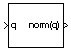# Quaternion Norm

Calculate norm of quaternion

• Library:
• Aerospace Blockset / Utilities / Math Operations

•## Description

The Quaternion Norm block calculates the norm for a given quaternion. Aerospace Blockset™ uses quaternions that are defined using the scalar-first convention. For the equations used for the quaternion and quaternion norm, see Algorithms.

## Ports

### Input

expand all

Quaternions in the form of [ q 0 , r 0 , ..., q 1 , r 1 , ... , q 2 , r 2 , ... , q 3 , r 3 , ...], specified as a quaternion norm or vector of quaternion norms.

Data Types: `double`

### Output

expand all

Quaternion norm or vector of quaternion norms in the form of [ norm (q), norm (r), ...].

Data Types: `double`

## Algorithms

The quaternion has the form of

`$q={q}_{0}+i{q}_{1}+j{q}_{2}+k{q}_{3}.$`

The quaternion norm has the form of

`$norm\left(q\right)={q}_{0}^{2}+{q}_{1}^{2}+{q}_{2}^{2}+{q}_{3}^{2}$`

 Stevens, Brian L., Frank L. Lewis. Aircraft Control and Simulation, Second Edition. Hoboken, NJ: Wiley–Interscience.